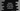# Python 3 program to find union of two lists using set## Python 3 program to find union of two lists using set :

In this tutorial, we will learn how to find the union of two lists using set in Python 3. To find the union of two lists, we don’t have any built-in methods. We are going to use ’set’ to find the union.

### Union :

Union of two list means it will contain all the elements of both list. For example, if one list contains (1,2,3,4) and another list contains (2,3,4,5) then the union of both list will be (1,2,3,4,5) .

### Set :

Set contains only unique elements. That means no two numbers will be same.

### Algorithm to find union of two lists :

1. First create two empty lists to store the user input values.

2. Ask the user to input_ total number of count_ for the first list.

3. Using a loop , take inputs for the first list.

4. Similarly, take total count and take all the inputs for the second list.

5. Now, append both lists and create one final list. This list may contain duplicate numbers. Convert this list to a ’set’ to remove the duplicate numbers.

6. Finally, Convert the ‘set’ to a ‘list’ and print out the result.

### Python 3 program :

``````first_list = []
second_list = []

#get total count for the first list
count_first_list = int(input("Enter total numbers of the first list : "))

#take inputs from the user for the first list
for i in range(1,count_first_list+1):
no = int(input("Enter : "))
first_list.append(no)

#get total count for the second list
count_second_list = int(input("Enter total numbers of the second list : "))

#take inputs from the user for the second list
for i in range(1,count_second_list+1):
no = int(input("Enter : "))
second_list.append(no)

#print first and second list
print("First list : ",first_list)
print("Second list : ",second_list)

#find union of both list
final_list = list(set(first_list + second_list))

#print the final list
print("Final list : ",final_list)
``````

### Sample Output :

``````Enter total numbers of the first list : 3
Enter : 1
Enter : 2
Enter : 3
Enter total numbers of the second list : 3
Enter : 2
Enter : 3
Enter : 4
First list :  [1, 2, 3]
Second list :  [2, 3, 4]
Final list :  [1, 2, 3, 4]
``````

### Explanation :

1. In the above example, we have fist combine both lists by using_ ’+’._

2. Next convert the total list to a set using ‘set(list)’ method.

3. It removed all the duplicate elements . Now convert this ’set’ to a ’list’ again by using ’list(set)’ method.

4. All the 1,2 and 3 steps are written in one line.

5. Final list is stored in variable ‘final_list’